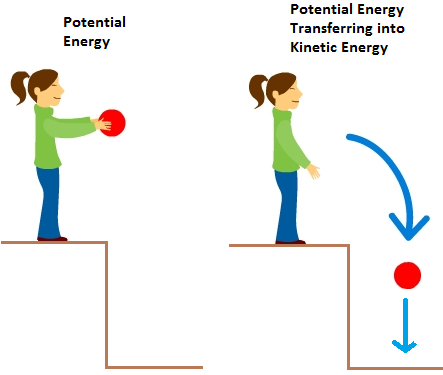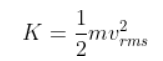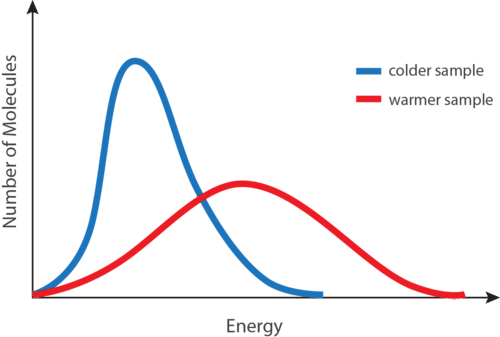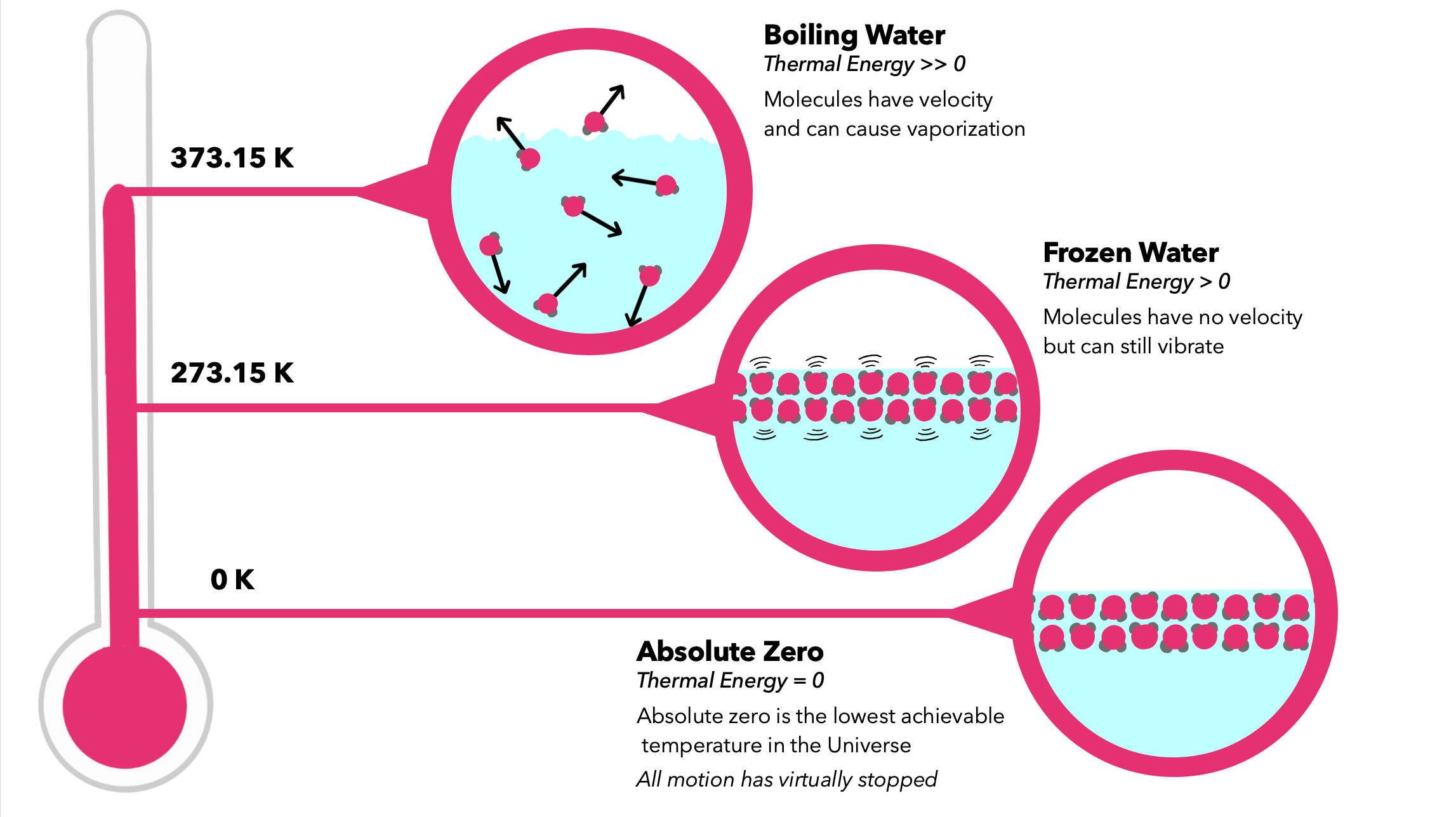# Average Kinetic Energy and Temperature Study Guide

INTRODUCTION

Have you ever ridden a roller coaster? At the top of the tracks, the roller coaster car does not have kinetic energy because it is at rest. As it goes down, its kinetic energy slowly increases with its speed. Similarly, when a person rides a bicycle, it possesses kinetic energy. As the bicycle’s velocity increases, so does its kinetic energy. A running person, a speeding bullet all have kinetic energy.

Kinetic energy (K.E.) is the energy possessed by an object in motion. Now, let’s understand kinetic energy and its dependence on other factors like temperature in more detail.Source

### KINETIC ENERGY AND TEMPERATURE

According to the kinetic-molecular hypothesis, a substance’s temperature is proportional to the average kinetic energy of its particles. When a substance is heated, some of the energy absorbed is kept inside the particles, while another energy accelerates particle motion. This is manifested as a rise in the material’s temperature.

When studying kinetic energy in gas molecules and its relationship with temperature, we generally define the term ‘average kinetic energy.

### AVERAGE KINETIC ENERGY:

The average kinetic energy of a gas molecule is defined as the product of half of the mass of each gas molecule and the square of the RMS speed. Mathematically, it’s as follows:m is the mass

vᵣₘₛ is the RMS velocity

K is the average kinetic energy

### RELATION BETWEEN AVERAGE KINETIC ENERGY AND TEMPERATURE:

The temperature of a substance is directly proportional to the average kinetic energy of the substance particles. Because the mass of these particles is constant, the particles must move faster as the temperature rises.

• Take two beakers, one with hot water and another with cold water.
• Add a few drops of any dye in both beakers.
• We observe that the dye particles in hot water show faster movement than those in cold water. The particles in both the beakers move at different speeds; they have different kinetic energies.
• We can conclude that the particles in the hotter body have a comparatively larger average K.E. than the colder one. In this way, the average K.E. of particles is indicated by their temperature.

We have seen the effect of increasing temperature on kinetic energy. Now let’s discuss the possibility of decreasing the temperature of the system and its effect on kinetic energy.Source

The kinetic energy of a particle decreases as we lower the system’s temperature. But imagine we keep decreasing the temperature and reach absolute zero. Then what happens to kinetic energy?

### ABSOLUTE ZERO:

• Absolute zero on the Celsius scale is −273.15℃. The Kelvin scale is based on absolute zero and starts at 0K.
• Absolute zero is where the kinetic energy of the particles is zero. That means all the motion between particles will cease.Source

## CONCLUSION:

• Kinetic energy is the energy possessed by an object in motion.
• The temperature of a substance is directly proportional to the average kinetic energy of the substance particles.
• Absolute zero is where the kinetic energy of the particles is zero.

### FAQs:

1. What happens at absolute zero?

At absolute zero, the thermodynamic system has the lowest temperature, and the K.E of the particles is zero.

2. What temperature is absolute zero in Fahrenheit?

Absolute zero corresponds to −459.67℉ in the Fahrenheit scale.

3. What is the lowest temperature achieved?

−273.144℃ is the lowest temperature that we achieved in the laboratory.

We hope you enjoyed studying this lesson and learned something cool about Average kinetic energy and Temperature! Join our Discord community to get any questions you may have answered and to engage with other students just like you! Don’t forget to download our App to experience our fun VR classrooms – we promise, it makes studying much more fun! 😎

## SOURCES:

1. Average kinetic energy and temperature. https://www.ck12.org/c/chemistry/average-kinetic-energy-and-temperature/lesson/Average-Kinetic-Energy-CHEM/. Accessed 17 Feb 2021.
2. Absolute zero. https://www.britannica.com/science/absolute-zero. Accessed 17 Feb 2021.
]]>